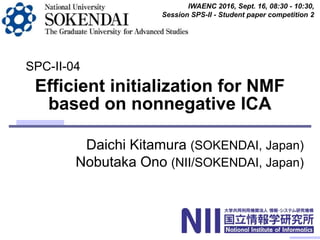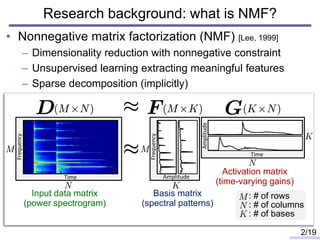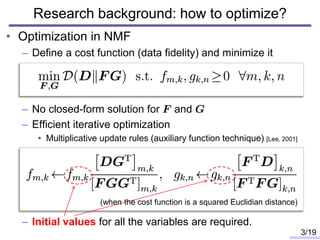Successfully reported this slideshow.

# Efficient initialization for nonnegative matrix factorization based on nonnegative independent component analysis

3

Share×
1 of 19
1 of 19

# Efficient initialization for nonnegative matrix factorization based on nonnegative independent component analysis

3

Share

Daichi Kitamura, Nobutaka Ono, "Efficient initialization for nonnegative matrix factorization based on nonnegative independent component analysis," The 15th International Workshop on Acoustic Signal Enhancement (IWAENC 2016), Xi'an, China, September 2016.

Daichi Kitamura, Nobutaka Ono, "Efficient initialization for nonnegative matrix factorization based on nonnegative independent component analysis," The 15th International Workshop on Acoustic Signal Enhancement (IWAENC 2016), Xi'an, China, September 2016.

## More Related Content

### Related Books

Free with a 14 day trial from Scribd

See all

### Related Audiobooks

Free with a 14 day trial from Scribd

See all

### Efficient initialization for nonnegative matrix factorization based on nonnegative independent component analysis

1. 1. Daichi Kitamura (SOKENDAI, Japan) Nobutaka Ono (NII/SOKENDAI, Japan) Efficient initialization for NMF based on nonnegative ICA IWAENC 2016, Sept. 16, 08:30 - 10:30, Session SPS-II - Student paper competition 2 SPC-II-04
2. 2. • Nonnegative matrix factorization (NMF) [Lee, 1999] – Dimensionality reduction with nonnegative constraint – Unsupervised learning extracting meaningful features – Sparse decomposition (implicitly) Research background: what is NMF? Amplitude Amplitude Input data matrix (power spectrogram) Basis matrix (spectral patterns) Activation matrix (time-varying gains) Time Time Frequency Frequency 2/19 : # of rows : # of columns : # of bases
3. 3. • Optimization in NMF – Define a cost function (data fidelity) and minimize it – No closed-form solution for and – Efficient iterative optimization • Multiplicative update rules (auxiliary function technique) [Lee, 2001] – Initial values for all the variables are required. Research background: how to optimize? 3/19 (when the cost function is a squared Euclidian distance)
4. 4. • Results of all applications using NMF always depend the initialization of and . – Ex. source separation via full-supervised NMF [Smaragdis, 2007] • Motivation: Initialization method that always gives us a good performance is desired. Problem and motivation 4/19 12 10 8 6 4 2 0 SDRimprovement[dB] Rand10 Rand1 Rand2 Rand3 Rand4 Rand5 Rand6 Rand7 Rand8 Rand9 Different random seeds More than 1dB Poor Good
5. 5. • With random values (not focused here) – Directly use random values – Search good values via genetic algorithm [Stadlthanner, 2006], [Janecek, 2011] – Clustering-based initialization [Zheng, 2007], [Xue, 2008], [Rezaei, 2011] • Cluster input data into clusters, and set the centroid vectors to initial basis vectors. • Without random values – PCA-based initialization [Zhao, 2014] • Apply PCA to input data , extract orthogonal bases and coefficients, and set their absolute values to the initial bases and activations. – SVD-based initialization [Boutsidis, 2008] • Apply a special SVD (nonnegative double SVD) to input data and set nonnegative left and right singular vectors to the initial values. Conventional NMF initialization techniques 5/19
6. 6. • Are orthogonal bases really better for NMF? – PCA and SVD are orthogonal decompositions. – A geometric interpretation of NMF [Donoho, 2003] • The optimal bases in NMF are “along the edges of a convex cone” that includes all the observed data points. – Orthogonality might not be a good initial value for NMF. Bases orthogonality? 6/19 Convex cone Data points Edge Optimal bases Orthogonal bases Tight bases satisfactory for representing all the data points have a risk to represent a meaningless area cannot represent all the data points Meaningless areas
7. 7. • What can we do from only the input data ? – Independent component analysis (ICA) [Comon, 1994] – ICA extracts non-orthogonal bases • that maximize a statistical independence between sources. – ICA estimates sparse sources • when we assume a super-Gaussian prior. • Propose to use ICA bases and estimated sources as initial NMF values – Objectives: • 1. Deeper minimization • 2. Faster convergence • 3. Better performance Proposed method: utilization of ICA 7/19 Number of update iterations in NMF Valueofcost functioninNMF Deeper minimization Faster convergence
8. 8. • The input data matrix is a mixture of some sources. – sources in are mixed via , then observed as – ICA can estimate a demixing matrix and the independent sources . • PCA for only the dimensionality reduction in NMF • Nonnegative ICA for taking nonnegativity into account • Nonnegativization for ensuring complete nonnegativity Proposed method: concept 8/19 Input data matrix Mixing matrix Source matrix … … Input data matrix PCA NMFInitial values NICA Nonnegativization …ICA bases PCA matrix for dimensionality reduction Mutually independent
9. 9. • Nonnegative ICA (NICA) [Plumbley, 2003] – estimates demixing matrix so that all of the separated sources become nonnegative. – finds rotation matrix for pre-whitened mixtures . – Steepest gradient descent for estimating Nonnegative constrained ICA 9/19 Cost function: where Observed Whitening w/o centering Pre-whitened Separated Rotation (demixing)
10. 10. • Dimensionality reduction via PCA • NMF variables obtained from the estimates of NICA – Support that , – then we have Combine PCA for dimensionality reduction 10/19 Rows are eigenvectors of has top- eigenvectors Eigenvalues High Low Basis matrix Activation matrix Rotation matrix estimated by NICA ICA bases Sources Zero matrix
11. 11. • Even if we use NICA, there is no guarantee that – obtained (sources) becomes completely nonnegative because of the dimensionality reduction by PCA. – As for the obtained basis (ICA bases), nonnegativity is not assumed in NICA. • Take a “nonnegativization” for obtained and : – Method 1: – Method 2: – Method 3: • where and are scale fitting coefficient that depend on a divergence of following NMF Nonnegativization 11/19 Correlation between and Correlation between and
12. 12. • Power spectrogram of mixture with Vo. and Gt. – Song: “Actions – One Minute Smile” from SiSEC2015 – Size of power spectrogram: 2049 x 1290 (60 sec.) – Number of bases: Experiment: conditions 12/19 Frequency[kHz] Time [s]
13. 13. • Convergence of cost function in NICA Experiment: results of NICA 13/19 0.6 0.5 0.4 0.3 0.2 0.1 0.0 ValueofcostfunctioninNICA 2000150010005000 Number of iterations Steepest gradient descent
14. 14. • Convergence of EU-NMF Experiment: results of Euclidian NMF 14/19 Processing time for initialization NICA: 4.36 s PCA: 0.98 s SVD: 2.40 s EU-NMF: 12.78 s (for 1000 iter.) Rand1~10 are based on random initialization with different seeds.
15. 15. • Convergence of KL-NMF Experiment: results of Kullback-Leibler NMF 15/19 PCA Proposed methods Processing time for initialization NICA: 4.36 s PCA: 0.98 s SVD: 2.40 s KL-NMF: 48.07 s (for 1000 iter.) Rand1~10 are based on random initialization with different seeds.
16. 16. • Convergence of IS-NMF Experiment: results of Itakura-Saito NMF 16/19 PCA Proposed methods x106 Processing time for initialization NICA: 4.36 s PCA: 0.98 s SVD: 2.40 s IS-NMF: 214.26 s (for 1000 iter.) Rand1~10 are based on random initialization with different seeds.
17. 17. Experiment: full-supervised source separation • Full-supervised NMF [Smaragdis, 2007] – Simply use pre-trained sourcewise bases for separation 17/19 Training stage , Separation stage Initialized by conventional or proposed method Cost functions: Cost function: Pre-trained bases (fixed) Initialized based on the correlations between and or
18. 18. • Two sources separation using full-supervised NMF – SiSEC2015 MUS dataset (professionally recorded music) – Averaged SDR improvements of 15 songs Experiment: results of separation 18/19 Separation performance for source 1 Separation performance for source 2 Rand10 NICA1 NICA2 NICA3 PCA SVD Rand1 Rand2 Rand3 Rand4 Rand5 Rand6 Rand7 Rand8 Rand9 12 10 8 6 4 2 0 SDRimprovement[dB] 5 4 3 2 1 0 SDRimprovement[dB] Rand10 NICA1 NICA2 NICA3 PCA SVD Rand1 Rand2 Rand3 Rand4 Rand5 Rand6 Rand7 Rand8 Rand9 Prop. Conv. Prop. Conv.
19. 19. Conclusion • Proposed efficient initialization method for NMF • Utilize statistical independence for obtaining non- orthogonal bases and sources – The orthogonality may not be preferable for NMF. • The proposed initialization gives – deeper minimization – faster convergence – better performance for full-supervised source separation 19/19 Thank you for your attention!

### Editor's Notes

• This is a background. This presentation treats NMF, which is nonnegative matrix factorization.
NMF is a dimensionality reduction with a nonnegative constraint, namely, all the decomposed components must be nonnegative, and the input matrix is represented by the linear combination of these nonnegative parts.
From this nature, the decomposed nonnegative parts tend to be meaningful features. For example, for acoustic signals, we often use a power spectrogram as the input data matrix.
In this case, by the NMF decomposition, we can extract some spectral patterns in the input spectrogram and their time-varying gains as nonnegative parts.
These patterns are often called basis vectors, and the gains are called activations.
From the nonnegative constraint, the decomposed parts implicitly tend to be sparse.
• In NMF, we define a cost function as a divergence between input data D and the decomposed model FG, and minimize it to find bases and activations under the nonnegative constraint.
Of course, there is no closed-form solution, but, efficient iterative update rules have been proposed.
These are the multiplicative update rules / when the cost function is a squared Euclidian distance.
However, this kind of optimization requires initial values for all the variables.
• And the problem is that / the results of all the applications using NMF / always depend on the initial values of F and G.
This graph shows an example of source separation performance based on a full-supervised NMF.
The vertical axis indicates the performance, and we tried with 10 random seeds.
The difference is more than 1 dB, so it strongly depends on the initial values. That’s the problem.
And our motivation is that / we want to develop an efficient initialization method / that always gives us a good performance.
• Some initialization techniques are already proposed for NMF, and they can be roughly divided into two types.
One is using random values, and the other one is without random values.
In this presentation, we only focus on the method without random values / because it is more stable than that using random values.
In this category, there have been two methods, PCA-based and SVD-based initializations.
Both of them are applying PCA or SVD to the data matrix D, then extract orthogonal bases that represent D.
Those can be used for the initial bases and activations.
• But the question is that, / are orthogonal bases really better for NMF? That’s problematic.
Because, from a geometric interpretation of NMF, it is reported that, the optimal bases in NMF are along the edges of a convex cone / that includes all the observed data points.
We here show that. Now we observed some data points. They must be in a positive orthant like this / because the data points are nonnegative, and the convex cone that includes all the data can be defined like this yellow tringle.
The optimal bases are along the edges of the cone because they are satisfactory for representing all the data.
The orthogonal bases can also represent them, but they have a risk to represent the meaningless area.
On the other hand, the tight bases cannot represent all the data points.
Therefore, we thought that / orthogonality might not be a good initial value for NMF.
• So what can we do from only the input data matrix? We here focused on ICA, independent component analysis.
Because ICA can extract non-orthogonal bases using statistical independence between coefficients, which are called sources.
Also, ICA can estimate sparse source if we assume a super-Gaussian prior.
Since NMF is also considered as a sparse decomposition, this feature may also be suitable.
So, in this presentation, we propose to use ICA bases and estimated sources as initial NMF values.
And the objectives are here, deeper minimization, faster convergence, and better performance for many applications. (ここで6分前後なら15分で終わる)
• This slide shows the concept of the proposed method.
We assume that the input data matrix is a mixture of some independent sources, namely, N sources in G, which are the activations, are mixed via ICA bases F, then observed as P1D.
Here, the matrix P1 is just a PCA matrix for the dimensionality reduction. I will show you the detail in the following slide.
Anyway, we assume the activations of each basis are mutually independent.
And this / is showing the process flow of the initialization, and here / is the proposed method.
First, we reduce the dimension of the input data matrix D by applying PCA. This is the orthogonal decomposition, but after that, we apply ICA. So it’s not a problem.
In this presentation, we apply nonnegative ICA to take the nonnegativity into account. This is also explained in the next slide.
Finally, nonnegativization is applied for ensuring complete nonnegativity, namely, we force all the estimates of NICA to be nonnegative.
• I briefly introduce nonnegative ICA. NICA estimates demixing matrix so that all of the separated sources become nonnegative.
This problem is equal to finding a rotation matrix W for pre-whitened mixtures.
Here we have an observed scatter x, and we apply whitening without data centering. Z is a whitened data.
Then, we just rotate z so that all the data are in a positive orthant. That’s corresponding to the demixing of sources.
And this is the cost function in NICA. That’s a minimization of the powers of remaining negative components.
The rotation matrix W can be estimated by the steepest gradient descent as shown in the bottom.
• Also, we combine PCA for only the use of dimensionality reduction.
P is a PCA matrix, so it has the eigenvectors of DD transpose, and P1 is the top-K eigenvectors.
We can easily derive a relationship between NMF variables, F and G, and NICA estimates W using P.
• Finally, we have to make the variables nonnegative at the end of initialization because, even if we use NICA, there is no guarantee that obtained F and G becomes completely nonnegative.
This is due to the dimensionality reduction.
So we take a nonnegativization for obtained F and G. We propose three types of nonnegativization.
The method 1 is just taking the absolute values of obtained F and G.
Method 2 is that we first take the absolute for bases, then, the nonnegative activations are calculated using correlations between the data D and F.
The method 3 is an inverse of method 2, where alpha is just a scale fitting coefficient calculated like this.
• We conducted an experiment.
This is the data matrix D. It’s a power spectrogram of the song obtained from SiSEC2015, and it includes vocals and guitar sounds.
The matrix size is here, and we set the number of bases to 60.
• This is a result of cost function in NICA.
We can confirm the convergence at around 2000 iterations.
• After the initialization, we iterate the NMF update rules.
We compared PCA-based and SVD-based initializations, random initialization, and the proposed method with 3 types of nonnegativization.
This graph shows the convergence of cost function in NMF.
Here we show random ones, SVD and PCA ones, and the proposed methods.
We can confirm that the proposed methods achieve faster and deeper convergence compared with the other methods.
Also, the second type of nonnegativization was the best performance in this experiment.
• Next, we confirmed the performance of proposed initializations in a full supervised source separation task.
In full-supervised NMF, we train the sample sound of each source in advance, and produce the sourcewise bases F1 and F2.
The training is a simple NMF, and we used various initialization methods here.
At the separation stage, F1 and F2 are used and fixed to separate the mixture, where the initial values of G1 and G2 are calculated based on the correlations between X and F1 or F2.
Therefore, the separation performance only depends on the initialization in the training stage.
• These graphs are the averaged results of 15 songs.
The left one is for source 1, and the right one is for source 2.
Although deeper minimization in NMF cost function does not directly ensure the separation performance, the proposed methods achieve more accurate separation compared with the other methods.
This might be because, for the separation using full-supervised NMF, the appropriate bases should be trained, and they should be minimum sufficient bases, namely, the bases along the edges of the data cone.
And, we expect that / the proposed method induces the bases to be such meaningful parts that are along the edges.